# Water Potential Problems Water Potential Water potential of

• Slides: 18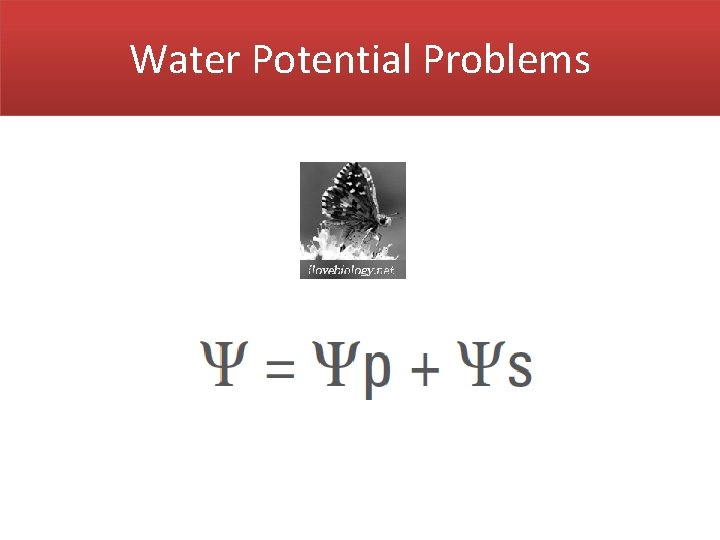Water Potential Problems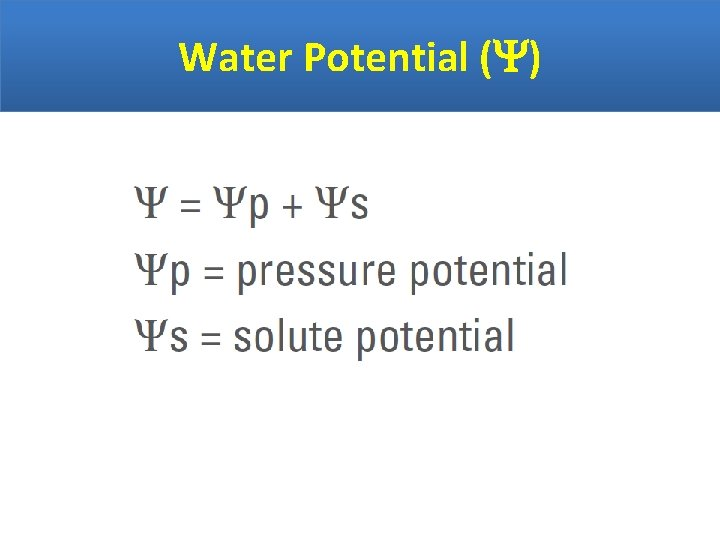Water Potential ( )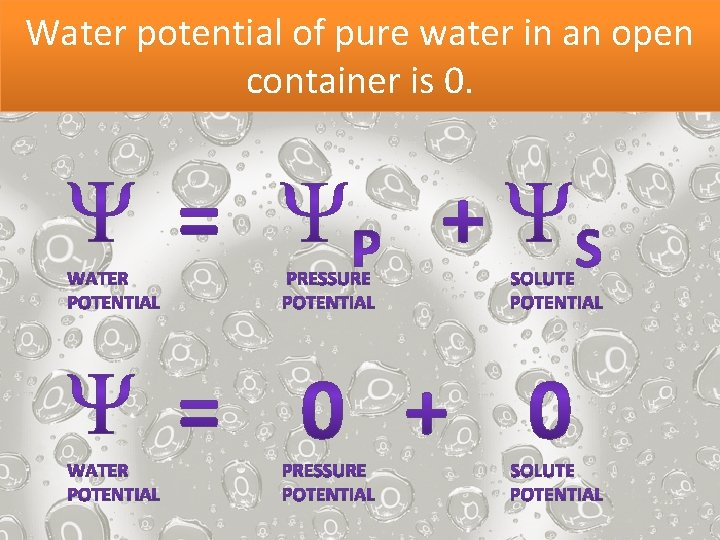Water potential of pure water in an open container is 0.Water will flow from an area of higher water potential to an area of lower water potential.Question 1: Which way will the water flow? Into the cell or out of the cell? = -2. 5 = -0. 5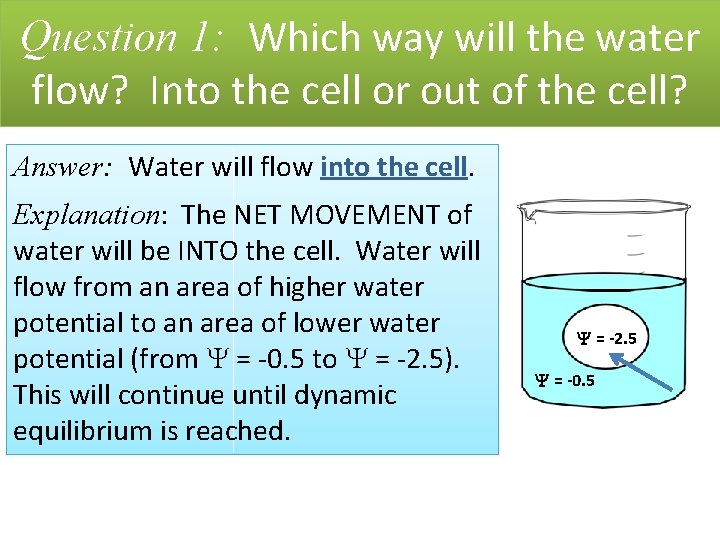Question 1: Which way will the water flow? Into the cell or out of the cell? Answer: Water will flow into the cell. Explanation: The NET MOVEMENT of water will be INTO the cell. Water will flow from an area of higher water potential to an area of lower water potential (from = -0. 5 to = -2. 5). This will continue until dynamic equilibrium is reached. = -2. 5 = -0. 5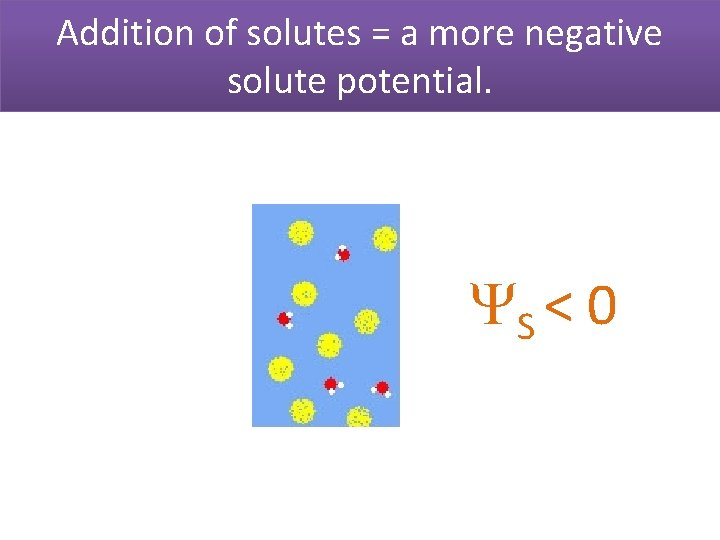Addition of solutes = a more negative solute potential. S < 0In an open container or in an animal cell, the pressure potential will be 0. P = 0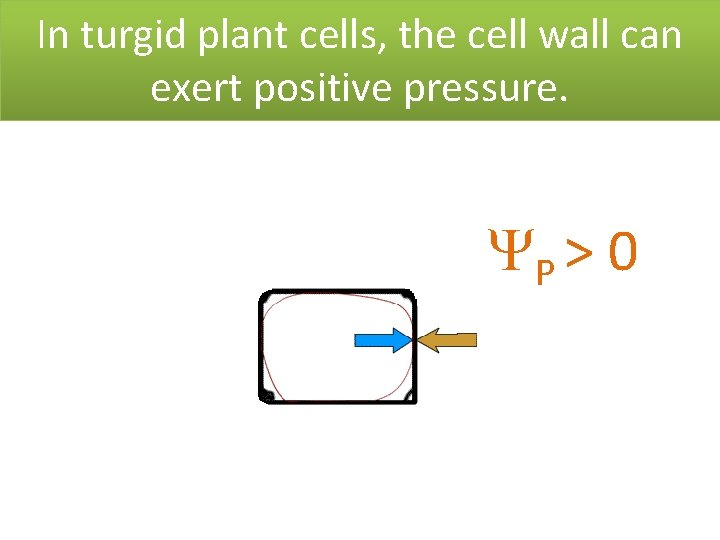In turgid plant cells, the cell wall can exert positive pressure. P > 0Solute Potential ( S)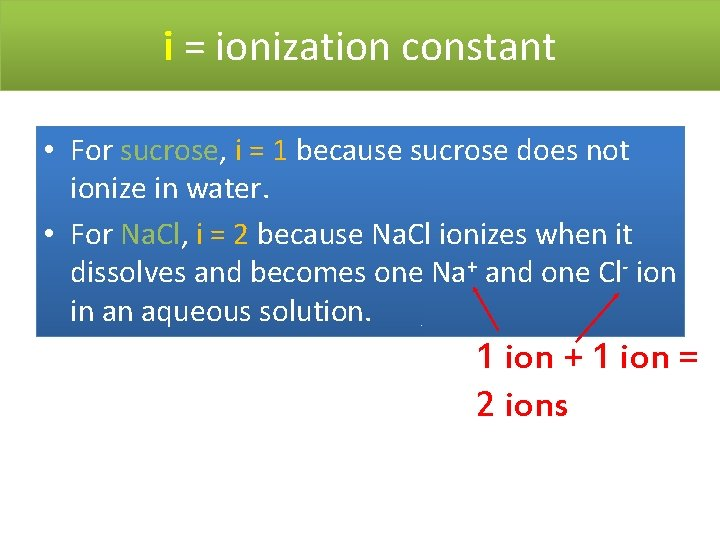i = ionization constant • For sucrose, i = 1 because sucrose does not ionize in water. • For Na. Cl, i = 2 because Na. Cl ionizes when it dissolves and becomes one Na+ and one Cl- ion in an aqueous solution. 1 ion + 1 ion = 2 ionsQuestion 2: For Mg. Cl 2, what would i be? Answer: i = 3 Explanation: Mg would ionize into 3 ions if dissolved in water: one Mg 2+ ion and two Clions.C is the molar concentration of the solution. Moles Solute Molarity = Volume of Solution M = moles L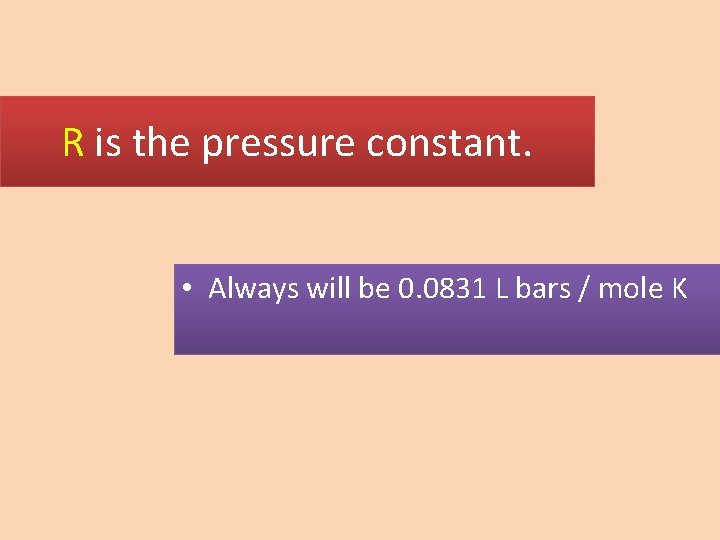R is the pressure constant. • Always will be 0. 0831 L bars / mole K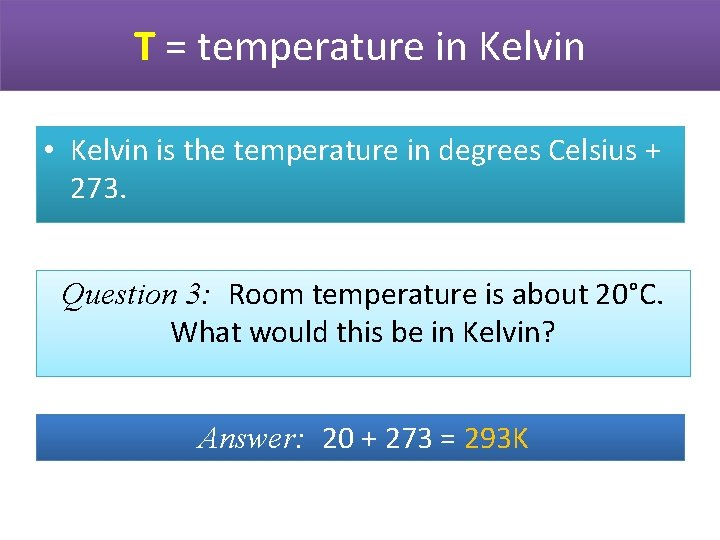T = temperature in Kelvin • Kelvin is the temperature in degrees Celsius + 273. Question 3: Room temperature is about 20°C. What would this be in Kelvin? Answer: 20 + 273 = 293 KExample 4: If a cell’s P = 3 bars and S = -4. 5 bars, what is the resulting ? = P + S Answer: = 3 bars + (-4. 5 bars) = -1. 5 barsQuestion 5: A cell with a = -1. 5 bars is placed in a beaker with a solution of = -4 bars. Will water flow into or out of the cell? Answer: Water will flow out of the cell from an area of higher water potential (-1. 5 bars) to an area of lower water potential (-4 bars).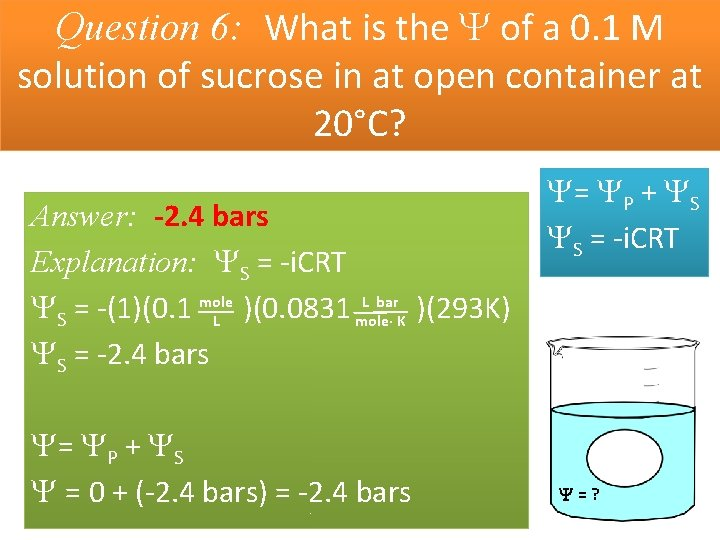Question 6: What is the of a 0. 1 M solution of sucrose in at open container at 20°C? Answer: -2. 4 bars Explanation: S = -i. CRT L bar S = -(1)(0. 1 mole )(0. 0831 )(293 K) L mole· K S = -2. 4 bars = P + S = 0 + (-2. 4 bars) = -2. 4 bars = P + S S = -i. CRT =?